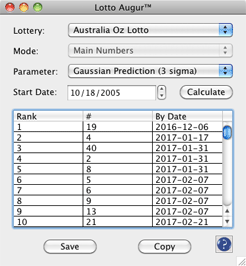## Lotto Augur### Overview

This function lets you check for various statistics to the selected lottery.

### How to Invoke

Use the menu item "Tools > Lotto Augur..." .

### Window Controls

Choose the lottery with which you wish to work.

If your lottery is a bonus-type lottery, you can choose from analyzing either the main numbers or the bonus number(s). If you lottery is not a bonus-type lottery, this menu will be ghosted and unavailable.

Depending on the lottery, you have up to twelve parameters from which to choose:

1. "Numbers Frequency" : this shows each number, how many times it has been drawn (in the "Count" ) column, and when the last time this number has been drawn (in the "Age" ) column.
2. "Most Common Numbers" : this lists, in descending order, the most common numbers that have been draw, how many times the number has been drawn, and the last time this number has been drawn.
3. "Least Common Numbers" : this lists, in ascending order, the least common numbers that have been draw, how many times the number has been drawn, and the last time this number has been drawn.
4. "Most Overdue Numbers" : this lists, in descending order the numbers that are the most overdue to be drawn, along with then number of times the numbers has been drawn, and the last time the number has been drawn.
5. "Most Common Pairs" : this lists the most common number pairs found in the drawings, followed by the number of times the pair have appeared. Because this counts occurences for every single two-number combination, it can take quite a while.
6. "Most Common Consecutive Pairs" : this lists the most common consecutive ('back-to-back') number pairs found in the drawings, followed by the number of times the pair have appeared. "Back-to-back" means two sequential numbers (in numerical order).
7. "Most Common Triplets" : this lists the most common number triplets found in the drawings, followed by the number of times the triplets have appeared. Because this counts occurences for every single three-number combination, it can take quite a while.
8. "Most Common Consecutive Triplets" : this lists the most common consecutive ('back-to-back-to-back') number triplets found in the drawings, followed by the number of times the triplets have appeared. "Back-to-back-to-back" means three sequential numbers (in numerical order).
9. "Odd versus Evens" : this shows all possible even-odd pairings, and how many times the pairings have occurred.
10. "Gaussian Prediction (1 sigma)": this parameters ranks, in order, which number is expected by the earliest date, calculated as 1 sigma.
11. "Gaussian Prediction (2 sigma)": this parameters ranks, in order, which number is expected by the earliest date, calculated as 2 sigma.
12. "Gaussian Prediction (3 sigma)": this parameters ranks, in order, which number is expected by the earliest date, calculated as 3 sigma.

#### "State Date" selector

Choose the starting date here. When you select a lottery in the Lottery dropdown menu, this date selector will default to the oldest (first) drawing.

#### "Calculate" button

This starts the calculation process.

#### "Save" button

Clicking this button will invoke a file selector, allowing you to save the results to a text file.

#### "Copy" button

This copies the results to the System Clipboard.